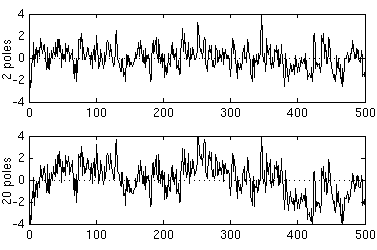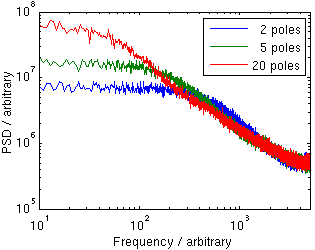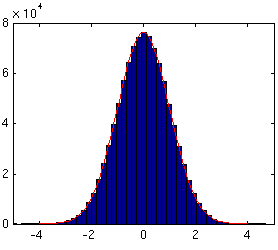# PinkNoise.java

A class for generating 1/fα pink noise

## Pink noise primer

Pink noise is a sequence of random numbers that have a specific form of frequency spectrum. Specifically, the power spectrum density (PSD) of the noise is proportional to 1/fα, where f is the frequency and 0 < α < 2. "Normal" pink noise has the parameter value α=1, but in many physical systems noise appears with different exponent values.

A more thorough explanation of pink noise is available on Wikipedia and on the page DSP generation of Pink (1/f) Noise.

## Methodology

In a recent project I required code that generated 1/f5/3 pink noise. The DSP generation of Pink (1/f) Noise page contains several pieces of code that generate 1/f pink noise, but I was unable to find any simple code that would allow specification of the exponent parameter α. I therefore wrote a new Java class for the purpose, and placed it here so others may benefit from it as well.

The class generates the noise by filtering white noise (plain pseudorandom numbers) with an infinite impulse response (IIR) filter. The filter is described by N. Jeremy Kasdin, Proceedings of the IEEE, Vol. 83, No. 5, May 1995, p. 822 equation (116).

The filter generates a pink noise sample xn from a white noise sample wn using the formula

xn = wn - a1 xn-1 - a2 xn-2 - a3 xn-3 - ...

where the coefficients are computed using

a0 = 1
ak = (k - 1 - α/2) ak-1 / k

This infinite sum is truncated with some number of terms, or poles. It was discovered that already 1–3 poles yield a good power spectrum at higher frequencies (read further for the properties and effect of the number of poles).

## Properties of the generated sequence

Since the IIR filter must be truncated, the power spectrum becomes constant below some limiting frequency. This limiting frequency is dependent on the number of poles used; the more poles are used the more low frequency components are included. A small number of poles results in noise centered at zero, while a large number of poles will have low frequency components and the sequence may deviate from zero for a long time. However, as any pink noise generated by filtering white noise, the resulting pink noise sequence is stationary, while true pink noise is non-stationary. This means that eventually the sequence will always return to zero. For most applications this is acceptable and even desirable, but some applications may require other generation techniques.

Below is a plot of two sequences of pink noise generated from the same random number sequence with two and twenty poles. This shows the qualitative difference that the number of poles has. The second figure shows the average power spectrum density of one hundred generated pink noise sequences with two, five and twenty IIR poles. The more poles are used, the lower the limiting frequency is.

The distribution of the values is very close to Gaussian with zero mean. However, the standard deviation is dependent on the number of poles used. In order to normalize the values to standard deviation one, generate a long sequence of pink noise with the same pole amount, calculate its standard deviation, and divide the generated samples by that value. Below is a histogram of a generated pink noise sequence with five poles, normalized to standard deviation one, along with the density function of the normal distribution. The two distributions are very close to one another.Click on a figure for a larger version.

## Usage

PinkNoise.java contains three constructors. The most general constructor allows defining the parameter α, the number of IIR poles to use and the Random object that will be used as a randomness source. The constructors with fewer arguments use the default values of α=1.0, 5 poles and a no-argument Random object.

The class supports generating pink noise for any 0 ≤ α ≤ 2. The special case α=0 corresponds to white noise (simple random numbers) and α=2 generates brown noise (integrated white noise).

After construction pink noise samples may be generated using the method nextValue(). The resulting values will be very close to the normal distribution with mean zero, but the standard deviation will be dependent on the number of poles used (see above).

The class also contains a main method, which demonstrates the usage and allows generating pink noise values from the command line.

For futher information, see the JavaDoc of PinkNoise.java.